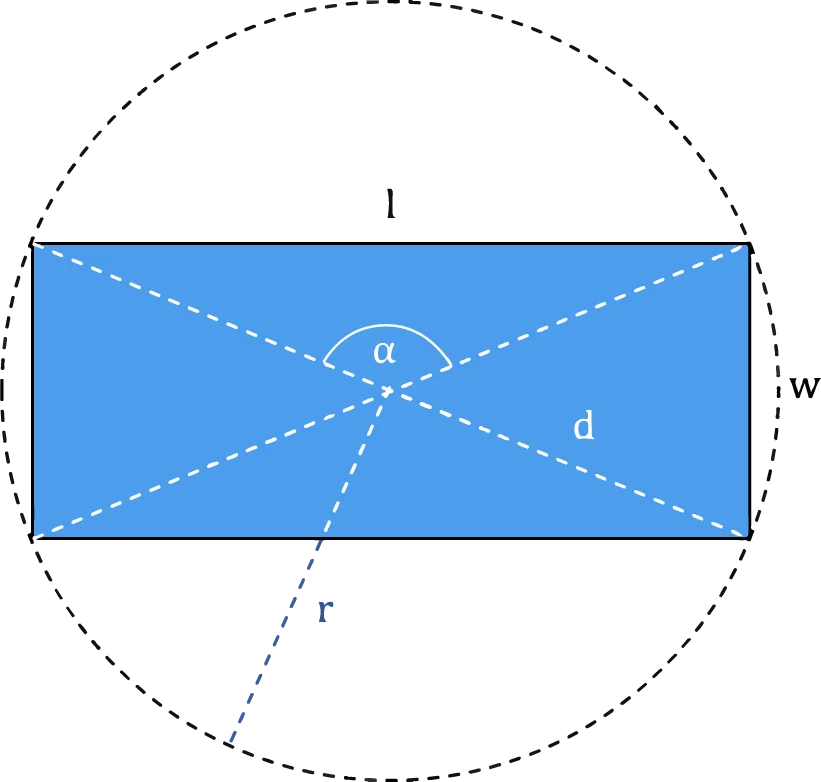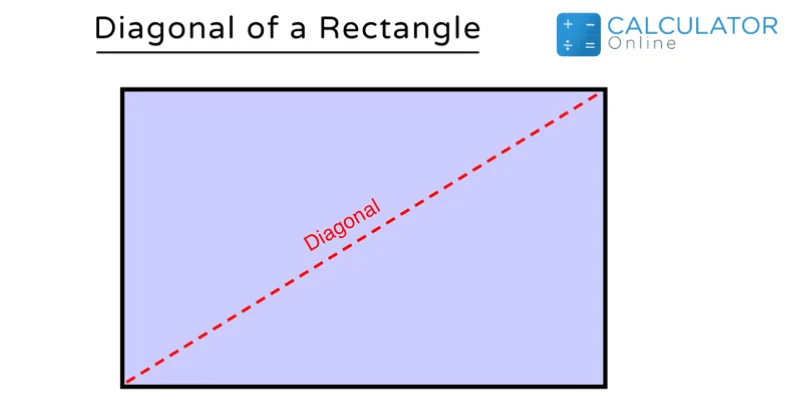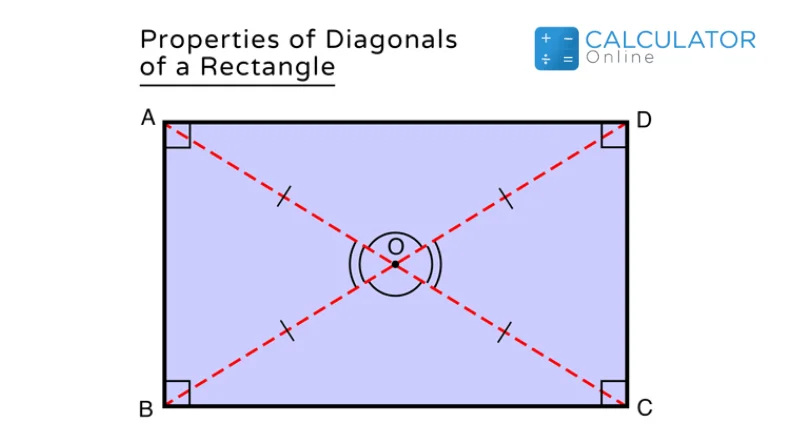Uh Oh! It seems you’re using an Ad blocker!

We always struggled to serve you with the best online calculations, thus, there's a humble request to either disable the AD blocker or go with premium plans to use the AD-Free version for calculators.

Or# Diagonal Calculator

Given

Longer side (I)

Shorter Side (w)

Area (A)

Perimeter (P)

Angle (α) between diagonals (α)Table of Content

Get the Widget!

Add this calculator to your site and lets users to perform easy calculations.

Feedback

How easy was it to use our calculator? Did you face any problem, tell us!

The diagonal calculator measures the diagonal, area, perimeter, and diagonal angle of a rectangle.  You just need to enter only two values to find the diagonal of the rectangle.

## Diagonal of a Rectangle:

A Rectangle is a quadrilateral having four angles and all the angles are 90 degrees. The opposite sides of the rectangle are parallel and equal in length. The adjacent sides of the rectangle are different in length, but the diagonals are equal in length and intersect in the middle point of the rectangle. We need to enter the length and width in the rectangle diagonal calculator to find the diagonal of the rectangle.### How to Find the Diagonal of a Rectangle?

We can find diagonal of a rectangle as:

Diagonal = √(l² + w²)

Where:

I = Length of the Rectangle

W = Width of Rectangle

#### Area of a Rectangle:

We can find the Area of the Rectangle by multiplying the length and width:

Area = l× w

#### The Perimeter of a Rectangle:

The perimeter of the rectangle can be measured by the given formula:

Perimeter = 2l + 2w=2(l+w)

We can find the diagonal of rectangle, area, and perimeter by the diagonal calculator.### Possibilities of Diagonal of Rectangle Measurements:

We can encounter various possibilities to find diagonal of a rectangle.

#### From Area and Length:

First, we have to find the length by the given formula:

l= A /w

Then,

P = 2l + 2w

Diagonal length of a rectangle = √(l² + w²)

#### From  Area and Width:

First, we have to find the width:

w= A / l

P = 2l + 2w

The diagonal measurement of a rectangle can be done as:

Diagonal = √(l² + w²)

#### From  Perimeter and Length:

w = (P – 2l) / 2

Area = l× w

Diagonal = √(l² + w²)

#### From  Perimeter and Width:

l= (P – 2w) / 2

Area = l× w

Diagonal = √(l² + w²)

### Practical Example:

Measure the length of the diagonal, area, and perimeter of a rectangle whose length is 4 units and width is 3 units.

Sol:

Length   = 4 units

Width   = 3 units

Substituting the values in the below equation

Diagonal = √(l² + w²)= √(4² + 3²)  = √(25²).

The rectangle diameter = 5 units.

Length of diagonal of a rectangle= 5 units.

Area of rectangle = l× w

Area of rectangle = 4× 3 = 12 unit²

Perimeter of the rectangle  = 2l + 2w

Perimeter of the rectangle  = 2(4) + 2(3)

The perimeter of the rectangle  = 8+ 6

The perimeter of the rectangle  = 14 units

### How to Use a Diagonal Calculator?

Stick  to the following steps for precise calculations by the diagonal measurement calculator

Input:

Enter any two of the given input and Hit the calculate button

Output:

• The diagonal of a rectangle
• The Area of a rectangle
• The Perimeter of a rectangle
• The circumcircle of a rectangle
• The angle of a rectangle

### References:

From the source of wikipedia.org: Circumscribed circles>,Triangles

From the source of wikihow.com: Diagonal,Length, and the Width